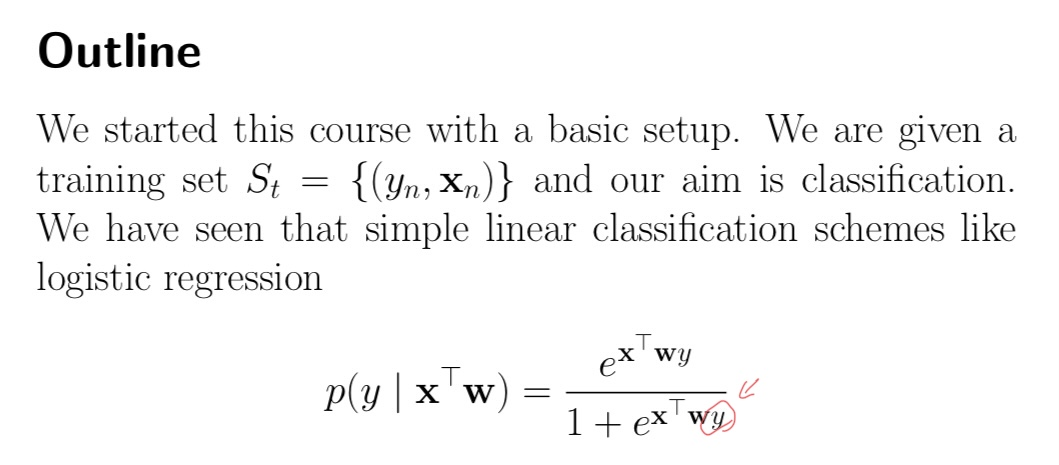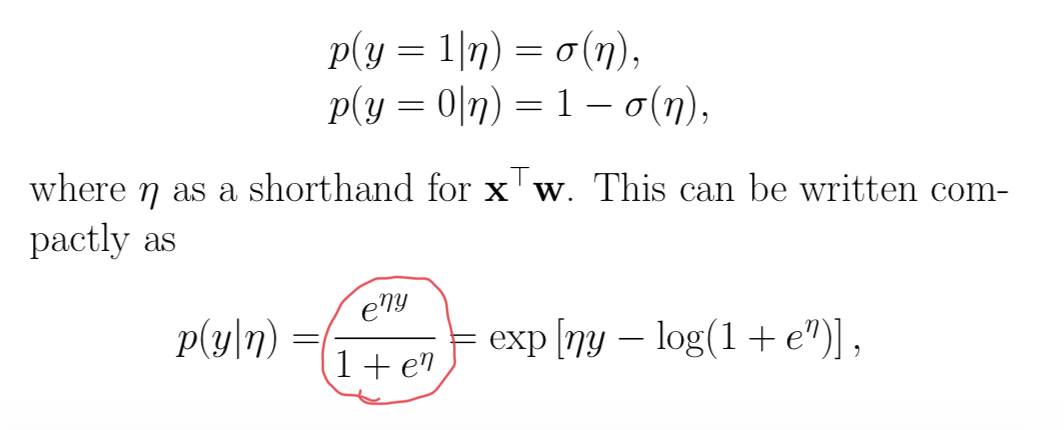### Logistic regression equation on the first page

Hello,

I wanted to ask if the equation in the photo is correct to have y in the power in the denominator or is it a mistake? Because I think it should be in the numerator power only.Hi,
I think the given equation is just sigmoid(xwy). In that case, there should be y in the denominator.
Best,
Semih

Thank you for your response, I just got confused between both equations since in case y=1 then the probability is sigmoid(XW) and if 0 then 1-sigmoid(Xw), as below.Perhaps it is because labels are {-1,1} in this case and sigmoid(x) + sigmoid(-x) = 1. So p(y=1 | wx) + p(y=-1 | wx) = 1.

Page 1 of 1